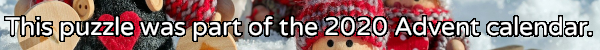mscroggs.co.uk
mscroggs.co.uksubscribe

## 18 DecemberThe expansion of $$(x+y+z)^3$$ is
$$x^3 + y^3 + z^3 + 3x^2y + 3x^2z + 3xy^2 + 3y^2z + 3xz^2 + 3yz^2 + 6xyz.$$
This has 10 terms.
Today's number is the number of terms in the expansion of $$(x+y+z)^{26}$$.
Tags: algebra

## Archive

Show me a random puzzle
▼ show ▼